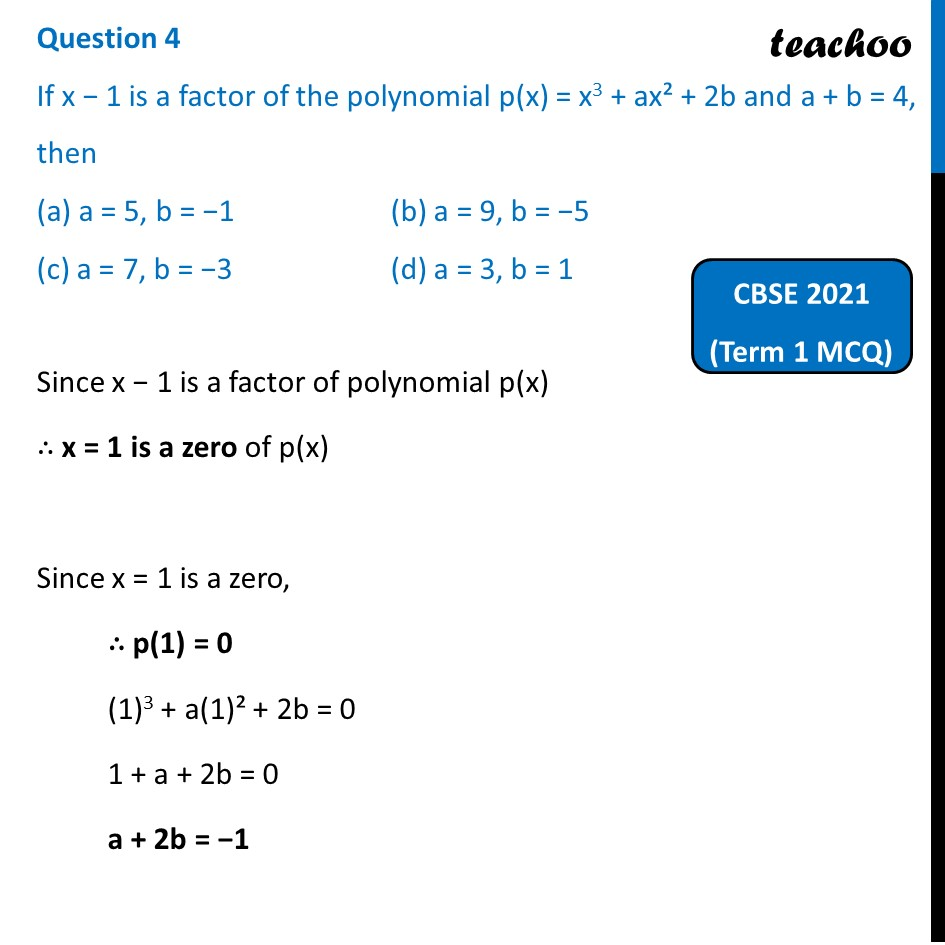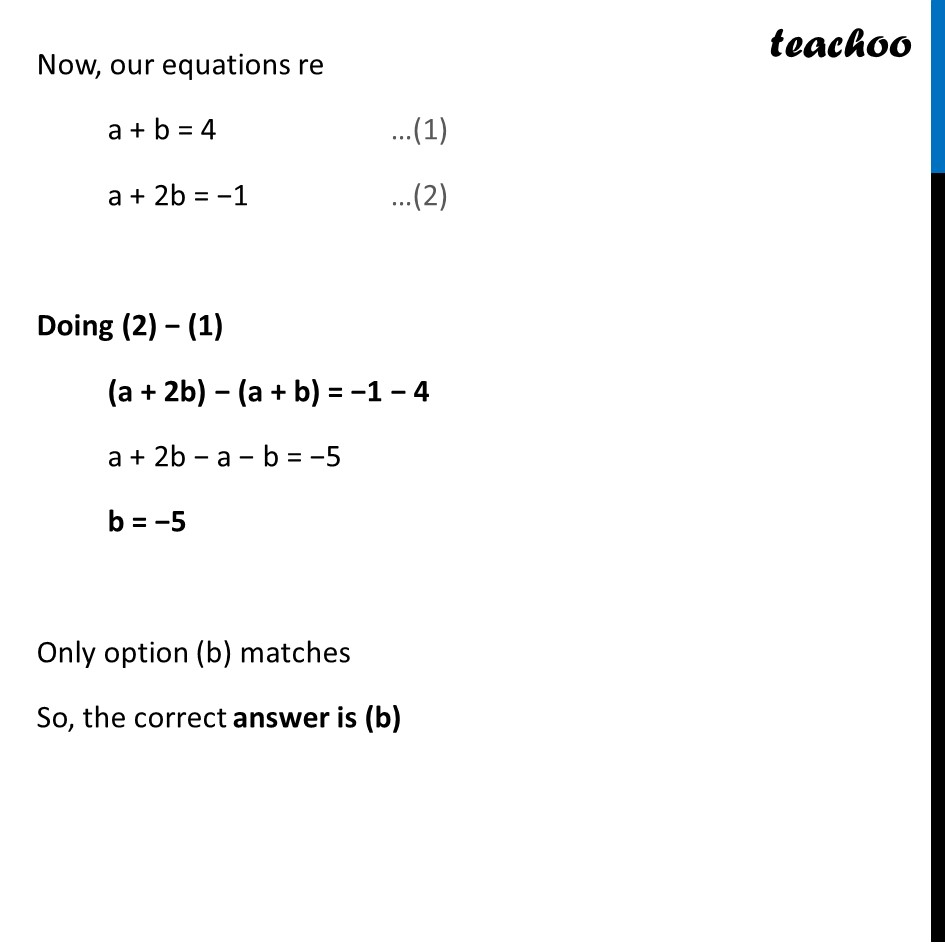Past Year MCQ (Maths Standard)

Chapter 2 Class 10 Polynomials
Serial order wise

## (d) a = 3, b = 1Learn in your speed, with individual attention - Teachoo Maths 1-on-1 Class

### Transcript

Question 4 If x − 1 is a factor of the polynomial p(x) = x3 + ax² + 2b and a + b = 4, then (a) a = 5, b = −1 (b) a = 9, b = −5 (c) a = 7, b = −3 (d) a = 3, b = 1 Since x − 1 is a factor of polynomial p(x) ∴ x = 1 is a zero of p(x) Since x = 1 is a zero, ∴ p(1) = 0 (1)3 + a(1)² + 2b = 0 1 + a + 2b = 0 a + 2b = −1 Now, our equations re a + b = 4 …(1) a + 2b = −1 …(2) Doing (2) − (1) (a + 2b) − (a + b) = −1 − 4 a + 2b − a − b = −5 b = −5 Only option (b) matches So, the correct answer is (b)